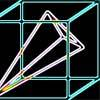#### You may also likeA box of size a cm by b cm by c cm is to be wrapped with a square piece of wrapping paper. Without cutting the paper what is the smallest square this can be?### Plane to See

P is the midpoint of an edge of a cube and Q divides another edge in the ratio 1 to 4. Find the ratio of the volumes of the two pieces of the cube cut by a plane through PQ and a vertex.### Four Points on a Cube

What is the surface area of the tetrahedron with one vertex at O the vertex of a unit cube and the other vertices at the centres of the faces of the cube not containing O?

# Interpenetrating Solids

##### Age 16 to 18 Challenge Level:

In mathematics, projections are often used to help understand the properties of 3 dimensional objects. Formally if an object is created from a set of points (x_n, y_n, z_n) then the projection in the z-direction, for example, will be the same set of points with the z coordinates squashed to zero (x_n, y_n, 0).

An obvious use of projections is in computer games where the 3D virtual world is shown on a flat screen.

It would be an interesting activity to attempt to construct real 3D models of the interpenetrated objects. Drinking straws would enable the creation of a framework and then the surfaces could be tiled with coloured paper cut to an appropriate size. It would be very interesting to receive photos of any such constructions!